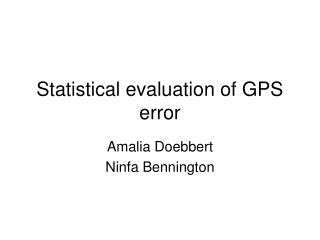DownloadDownload PresentationStatistical evaluation of GPS error

# Statistical evaluation of GPS error

Download Presentation## Statistical evaluation of GPS error

- - - - - - - - - - - - - - - - - - - - - - - - - - - E N D - - - - - - - - - - - - - - - - - - - - - - - - - - -
##### Presentation Transcript

1. Statistical evaluation of GPS error Amalia Doebbert Ninfa Bennington

2. Problem Assumed uncertainties in GPS systems: • sHH < 10 m • sDGPS < 1 m • Big questions: • Do uncertainties we observe in real data agree with these estimates? • Are the distributions of these data Gaussian?

3. Data used Field exercise 26 April, 2008 DGPS: • 3 Trimble rcvrs • 10 sec. epochs HH GPS: • 3 HH rcvrs • ~10 min. tracks • Variable epochs

4. Methods

5. HH absolute position histograms m =43.0709º s =3.19 m

6. DGPS relative position histograms m =1.1574 m s =.1286 m

7. Methods

8. Z-distribution (HH) • Z=(x-m)/s • Hist(Z)

9. Z-distribution (DGPS) • Z=(x-m)/s • Hist(Z)

10. Methods

11. c2 (Goodness of fit) c2 = S[(O-E)/s]2

12. Results (DGPS)

13. Results (DGPS)

14. Results (DGPS)

15. Results (HH)

16. Results (HH)

17. Results (HH)

18. Conclusions • Positions from DGPS better fit to Gaussian distribution than HH • Assuming Gaussian distribution: • sHH between ±0.21 m and ±5.06 m • sDGPS,vert < 0.2839 m & sDGPS,NorE < 0.1822 m • DGPS positioning more precise than HH positioning • Both DGPS and HH have uncertainties w/in estimated uncertainty